# The ketchup

If 3 1/4 of tomatoes are needed to make 1 bottle of ketchup. Find the number of tomatoes required to make 4 1/5 bottles

n =  13.65

### Step-by-step explanation: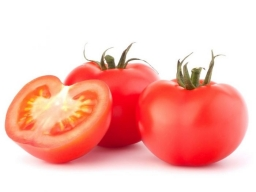Did you find an error or inaccuracy? Feel free to write us. Thank you!Tips to related online calculators
Need help to calculate sum, simplify or multiply fractions? Try our fraction calculator.

## Related math problems and questions:

• Fractions and mixed numerals(a) Convert the following mixed numbers to improper fractions. i. 3 5/8 ii. 7 7/6 (b) Convert the following improper fraction to a mixed number. i. 13/4 ii. 78/5 (c) Simplify these fractions to their lowest terms. i. 36/42 ii. 27/45 2. evaluate the follow
• Fraction of a NumberIf 1/2 of 1/3 of 1/4 of 1/5 of a number is 2.5. what is the number?
• Muffins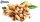One batch of walnut muffins uses 1 1/3 cups of walnuts. How many cups of walnut are needed to make 3 3/4  batches of muffins?
• FlourKim needs 3/4 cup of flour to make 12 cookies. How much flour would she need to make 60 cookies?
• A bottle 2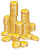A bottle contain 255 coins 1/3 of the coins are £1 cons 110 of the coins are 50p coins the rest of the coins are 20p coins. What is the total value of the coins contained in the bottle.
• Evaluate expressionEvaluate expression using BODMAS rule: 1 1/4+1 1/5÷3/5-5/8
• Jim collected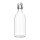Jim collected 5 bins of glass bottles to recycle. Lucia collected 3 1/5 times as many bins as Jim. How many bins of bottles did Lucia collect?3 3/4 + 2 3/5 + 5 1/2 Show your solution.
• Numbers divisionWith what number should be divided mixed number 2 3/4 to get 11/12?
• Divide 11Divide the product of 4 and 5/8 by 1 1/2. Write your answer as a mixed number.
• The sum 12The sum of 3 mixed numbers is 20 13/15. two of the numbers are 6 1/3 and 7 5/6. what is the third number?
• Unknown number4/5 of a number is 276. what is 2/3 of the same number?
• The buildingThere are 45 people living in building, out of these 3/5 are men,2/5 are women . find the number of men and women in the building.
• Trees3/5 trees are apples, cherries are 1/3. 5 trees are pear. How many is the total number of trees?
• Unknown number 6Determine x if 1/6 of x is equal to 2/5 of the number 24.
• Find unknown 2Find unknown denominator: 2/3 -5/? = 1/4
• Land cost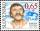The land is worth 33295 CZK. 1st owner has 5/15, 2nd owner has 4/15, 3rd owner has 1/5 4th owner has 1/5. How much CZK each of them will receive?